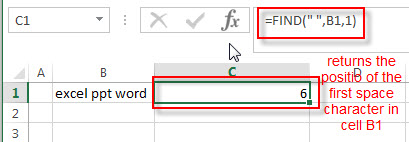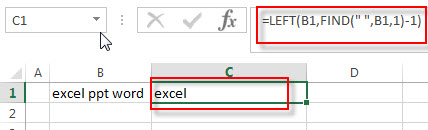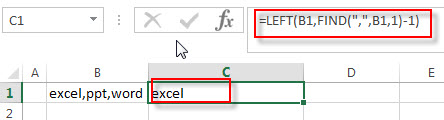# How to extract text before first comma or space

This post explains that how to extract a substring before the first specific character, such as: comma or space character. How to get text before the first comma in a text string using a formula in excel.

## Extract text before first comma or space

If you want to extract text before the first comma or space character in cell B1, you can use a combination of the LEFT function and FIND function to create a formula as follows:

`=LEFT(B1,FIND(" ",B1,1)-1)`

Let’s see how this formula works:

= FIND(” “,B1,1)The FIND function returns the position of the first empty string in a text string in Cell B1, it returns 6. And the returned value goes into the LEFT function as its num_chars argument.

=LEFT(B1,FIND(” “,B1,1)-1)The LEFT function extracts a specified number of the characters from a text string in Cell B1, starting from the leftmost character.

If you want to extract a substring before the first comma in a text string in Cell B2, you just need to change the empty string to comma string in the above formula, like this:

=LEFT(B1,FIND(“,”,B1,1)-1)### Related Formulas

• Extract Text between Parentheses
If you want to extract text between parentheses in a cell, then you can use the search function within the MID function to create a new excel formula…
• Extract Text between Brackets
If you want to extract text between brackets in a cell, you need to create a formula based on the SEARCH function and the MID function….
• Extract Text between Commas
To extract text between commas in Cell B1, you can use the following formula based on the SUBSTITUTE function, the MID function and the REPT function…..
• Split Text String by Line Break in Excel
When you want to split text string by line break in excel, you should use a combination of the LEFT, RIGHT, CHAR, LEN and FIND functions. The CHAR (10) function will return the line break character, the FIND function will use the returned value of the CHAR function as the first argument to locate the position of the line break character within the cell B1.…
• Split Text and Numbers
If you want to split text and numbers, you can run the following excel formula that use the MIN function, FIND function and the LEN function within the LEFT function in excel. And if you want to extract only numbers within string, then you also need to RIGHT function to combine with the above functions..…

### Related Functions

• Excel LEFT function
The Excel LEFT function returns a substring (a specified number of the characters) from a text string, starting from the leftmost character.The syntax of the LEFT function is as below:= LEFT(text,[num_chars])….
• Excel FIND function
The Excel FIND function returns the position of the first text string (sub string) within another text string.The syntax of the FIND function is as below:= FIND(find_text, within_text,[start_num])…
Related Posts

Abbreviate Names Or Words in Excel

As an MS Excel user, you might have come across a task where you need to abbreviate different names or words, and there are also possibilities that you might have done this task manually by assuming that there isn't any ...

Filter with Multiple Criteria

If you are an valid MS Excel user, you have probably come across a situation where you wanted to filter the data in a separate table with complex criteria. You could do this task manually, which is also acceptable while ...

Extract substring In Excel

This post will guide you how to use Excel's MID function is a quick and easy way to extract pieces from your text. Use the Excel formula to extract a substring with MID. Note: If you want to extract just ...

How to Split Cells by the First Space in Texts in Excel

Sometimes we may use space to separate texts to different groups in one cell, if we want to split this cell to multiple columns refer to space, we can implement this via ‘Text to Columns’ feature. But is there any ...

How to Convert Uppercase to Lowercase Except the First Letter in Excel

In excel, words can be entered in uppercase, lowercase or mixed. If we want to convert all uppercase to lowercase (except the first letter in some situations), we can use formula with some letter convert related functions to convert them ...

How to Remove Middle Name from Full Name in Excel

When we get a list of full names, we can remove the middle name for short in some cases. As the middle names are different among the full name list so we cannot replace them by space directly in excel. ...

How to Convert Text to Time in Excel

This post will guide you how to convert time string to time in Excel. Assuming that you have a list of text string in your worksheet, and you wish to convert these time string to standard time format, how to ...

How to Extract Initials From a Name in Excel

This post will guide you how to get initials from a given name using a formula in Excel. How do I extract initials from names in Excel 2013/2016. Extract Initials from a Name Using a Formula Extract Initials from a ...

How to Remove Last or Trailing Commas in Excel

This post will guide you how to remove trailing commas from cell in Excel. How do I remove comma character at the end of a cell with a formula in Excel 2013/2016. Remove Trailing Commas if you want to remove ...

How to Filter Cells Starts with Number or Letter in Excel

This post will guide you how to filter values starts with number or letter in one single cell in Excel. How do I filter in a list those that begin with number or letter using a formula in Excel. Filter ...

Sidebar